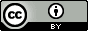## Monday, December 2, 2013

### Notes on fugacity and activity

This is one of those "note to self" posts where I try to get my head around a concept, this time fugacity and activity for a gas.

$dG=Vdp-SdT \implies dG=Vdp \text{ if } dT=0$
$$G(p)=G^\circ+\int_{p^\circ}^{p}Vdp$$
If the gas is ideal, i.e. for one mole $V=RT/p$, then
$$G(p)=G^\circ+RT\int_{p^\circ}^{p}\frac{dp}{p}=G^\circ+RT\ln\frac{p}{p^\circ}$$
For $A\rightleftharpoons B$
$$G(p_B)-G(p_A)=0 \implies \frac{p_B}{p_A}=e^{-\Delta G^\circ/RT}$$
What about a real gas where $V\neq RT/p$?  We introduce the fugacity $(f)$ for which $V=RT/f$ so that
$$G(p)=G^\circ+RT\ln\frac{f}{p^\circ} \text{ and } \frac{f_B}{f_A}=e^{-\Delta G^\circ/RT}$$
To determine $f$:
$$\int_{p'}^{p} (V-V_{ideal})dp=RT\ln\left(\frac{f}{f'}\cdot \frac{p'}{p}\right) = RT\ln\left(\frac{f}{p}\cdot \frac{p'}{f'}\right)$$
Gases approach ideality at low pressure: $f'/p'\rightarrow 1$ as $p\rightarrow 0$ so:
$$\ln\left(\frac{f}{p}\right)=\ln(\phi)=\frac{1}{RT}\int_{0}^{p} (V-V_{ideal})dp$$
So for sticky non-ideal gases for which $V<V_{ideal}$ the fugacity coefficient $\phi$ is less than 1.  So even though $V=RT/f$ don't confuse $f$ with $p_{ideal}: f<p<p_{ideal}$ for a given number of gas particles.

Finally the relationship between fugacity and activity ($a$) is
$$a=\frac{f}{p^\circ}$$.